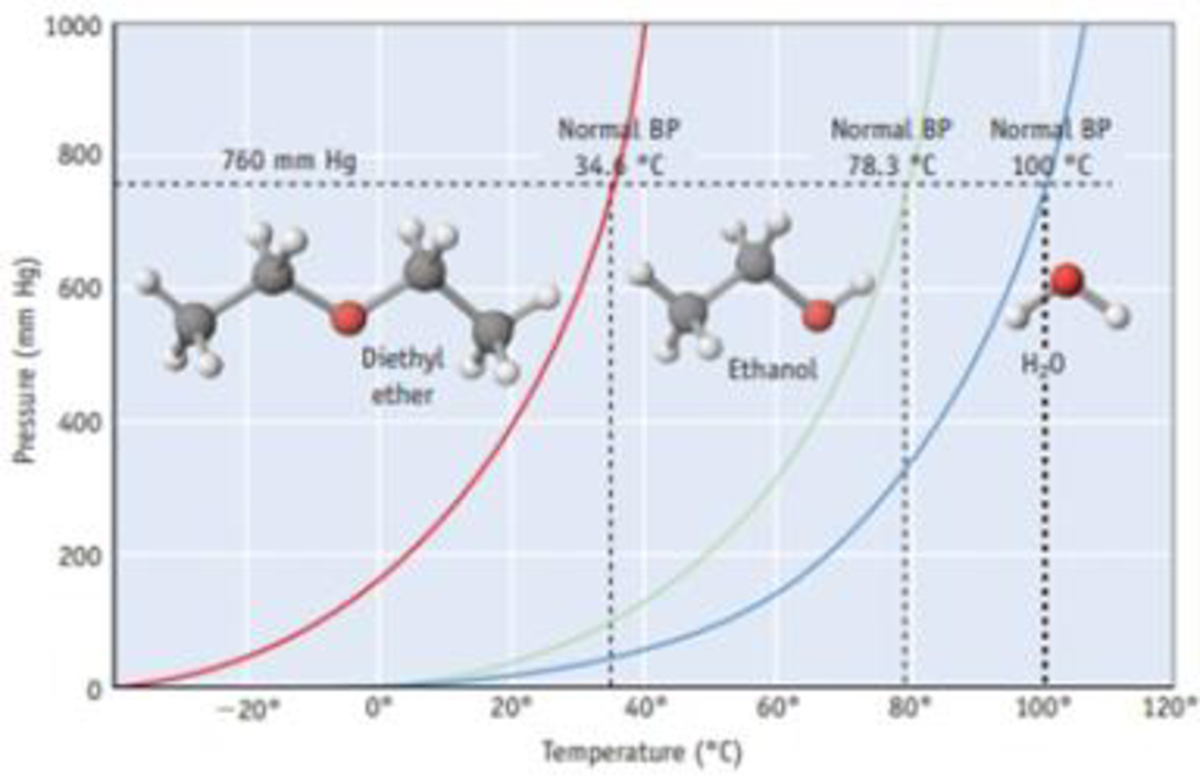Chapter 11, Problem 15PS

Chapter
Section
Textbook Problem

Assume you seal 1.0 g of diethyl ether (Figure 11.12) in an evacuated 100.-mL flask. If the flask is held at 30 °C, what is the approximate gas pressure in the flask? If the flask is placed in an ice bath, does additional liquid ether evaporate, or does some ether condense to a liquid?Figure 11.12 Vapor pressure curves for diethyl ether [(C2H3)2O], ethanol (C2H5OH), and water. Each curve represents conditions of T and P of which the two phases, liquid and vapor, are in equilibrium. These compounds exist as liquids for temperatures and pressures to the left of the curve and as gases under conditions to the right of the curve. (See Appendix G for vapor pressures for water of various temperatures.)

Interpretation Introduction

Interpretation: The approximate gas pressure of ether at 30oC should be determined. The additional ether is whether evaporated or condensed when placed in an ice bath should be determined

Concept Introduction: Vapor pressure is nothing but the pressure of a vapor in contact with its liquid or solid form.

When a liquid and vapor are in equilibrium the pressure exerted by the vapor is called the equilibrium vapor pressure.

The ideal gas equation is,

PV=nRTwhere, P=Pressure V=Volume n=Number of moles R=Gasconstant T=Temperature

From its given mass is,

Number of moles=GivenmassMolecularmass

Explanation

Temperature=25oC=(25+273)=303KVolume=100mL=0.10L

The figure is given,

From the figure, the vapor pressure of dimethyl ether at 300C is 590mmHg.

We know,PV=nRT

Rearranging the equation,

n=(590mmHg760mmHg)×0.1L(0.0821atmLmolK)×303K=0.0033mol

From its given mass is,

Number of moles=GivenmassMolecularmass

Rearranging the equation,

Givenmass=Number of moles×Molecularmass=0

Still sussing out bartleby?

Check out a sample textbook solution.

See a sample solution

The Solution to Your Study Problems

Bartleby provides explanations to thousands of textbook problems written by our experts, many with advanced degrees!

Get Started

The best way to control salt intake is to cut down on processed and fast foods. T F

Nutrition: Concepts and Controversies - Standalone book (MindTap Course List)

Speed is a(n) ___ quantity. (2.2)

An Introduction to Physical Science

1-53 Which states of matter have a definite vo1ume?

Introduction to General, Organic and Biochemistry

What is the most common type of star?

Horizons: Exploring the Universe (MindTap Course List)